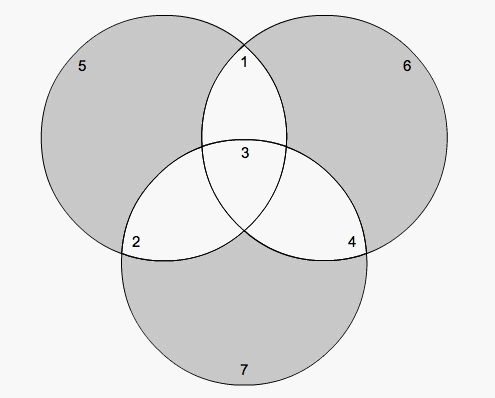# Lecture 5: Hamming Codes

Let's pause for a moment here and think about the strengths and weaknesses of the codes that we have discussed so far, the repeating code and the parity check code. Here are some salient questions: How many bits are in the codeword? How long a message (in bits) is sent with this codeword? How many error bits in transmission of the codeword can the code detect? What is the Hamming distance? How many can it correct? Try to answer these questions for both the repeating code and the parity check code.

As you can can see, the repeating code is pretty unwieldy, since to send a one bit message you send an n bit codeword. That is an awful lot of information to send for not much message. The parity check code has different flaws. While it doesn't require sending an ubsurly long codeword for a quite short message, the parity check code can only detect an odd number of errors, make two of them and it doesn't know any better. This essentially means that it can only detect one error. (What does this have to do with the Hamming distance for the partiy check code?) Furthermore, the parity check code can't correct any errors at all, it only knows that the wrong nmber of 1s are present in the codeword.

So we really would like something more of a middle ground, not too long a codeword relative to the message, that can both detect and correct some errors. One of the possible answers to this question is the Hamming code. Hamming codes are a large class of codes, but we will only talk about one example, the (7,4) Hamming code, which was introduced by Hamming in 1950 (this is the same Hamming of Hamming distance fame from the last lecture). This code turns a 4 bit message into a 7 bit codeword that contains the 4 original message bits plus 3 more parity check bits, which we will get by applying the parity check code to various bits of the original message. Hamming invented this code for punch card computer systems which aren't used very often anymore. However, Hamming codes are still used in communication between the earth and satillites that we send to outerspace.

Here is how we figure out the parity check bits: we put the message in the parts of the three circles labeled 1 to 4 (the first bit of the message in the region labeled 1 and the last in the region labeled 4).Then, we apply the parity check code to each of the circles, adding a 1 as nesscary in the remaining compartment of the circle to make sure that there is an even number of 1s in the circle. We then send the codeword that is the digits in their labeled order. For example, the message 1000 will be encoded as 1000110. What will the encoding of the message 0110 be? 0010? 1011? 1111?

Now lets look at what this code will do in the way of error detection and correction. When we receive a message, we put in back in our picture of the circles, with the parity bits and message bitsi n the right place. Do each of the circles have an even number of 1s in the recieved codeword 0100110? Does this suggest an error? Now that you can see that there is an error somewhere in recieved codeword, by checking each of the parity bits, can you make a suggestion about where that error is likely to be? Can you correct it?

There is an applett for encoding and decoding the (7,4) Hamming code here that was devleoped by engineers at Caltech. Try generating a message (click the squares once to get a 0, twice to get a 1). Then encode your message and add erasures or errors. How many bits can you correct? Does the answer depend on whether you inserted erasures or errors? Does it depend on where your erasures or errors were?

Hints and Solutions:
• What does this have to do with the Hamming distance for the partiy check code? The parity check code has Hamming distance one, so an error in more than one bit makes the codeword with errors into another codeword, so we can't correct.
• What will the encoding of the message 0110 be? 0110010. 0010? 0010111 1011? 1011010. 1111? 1111111.
• Do each of the circles have an even number of 1s in the recieved codeword 0100110? Does this suggest an error? No, the top right circle has one 1 in it, which isn't an even number, suggesting an error in that circle, the same is true for the bottom circle.
• Now that you can see that there is an error somewhere in recieved codeword, by checking each of the parity bits, can you make a suggestion about where that error is likely to be? Can you correct it? Since the error is in the upper right circle and also the bottom one, but not in the upper left circle, this suggests it is in the only region that is in the two circles where the parity check doesn't come out that aren't shared with the circle where the parity check does work, that is, the error is in bit four. Notice that if we change bit four from a 0 to a 1, that parity checks all work, which leads us to believe that this is the codeword that we should have recieved.

This work was made possible through a grant from the National Science Foundation.